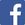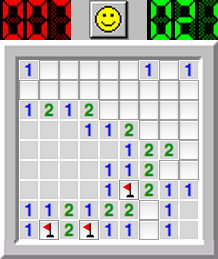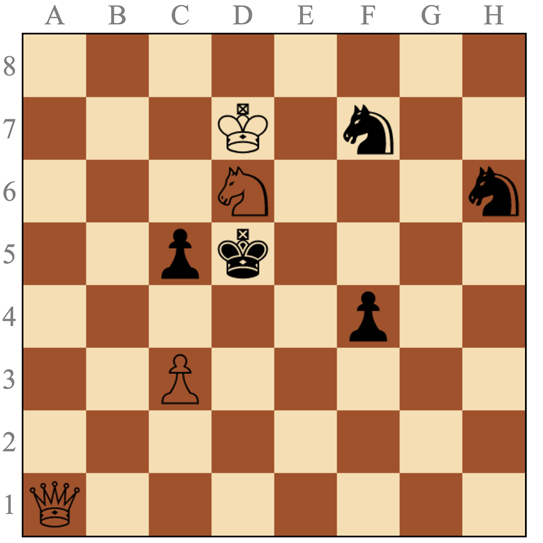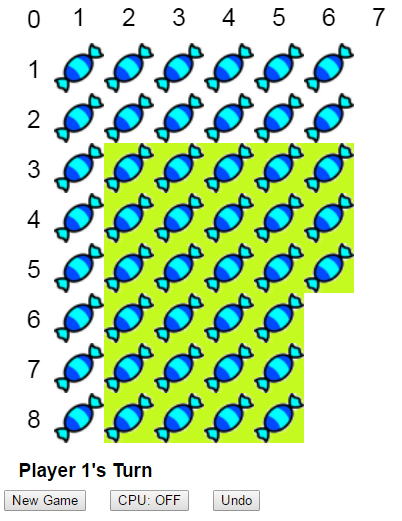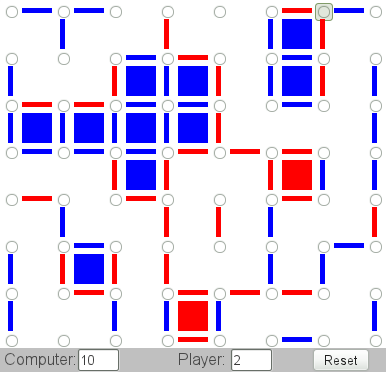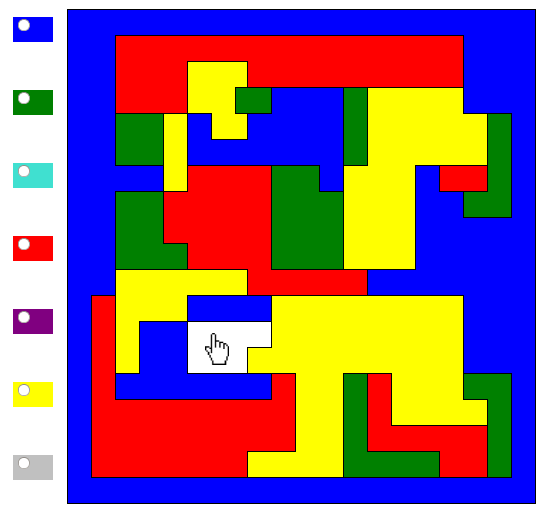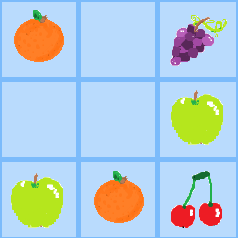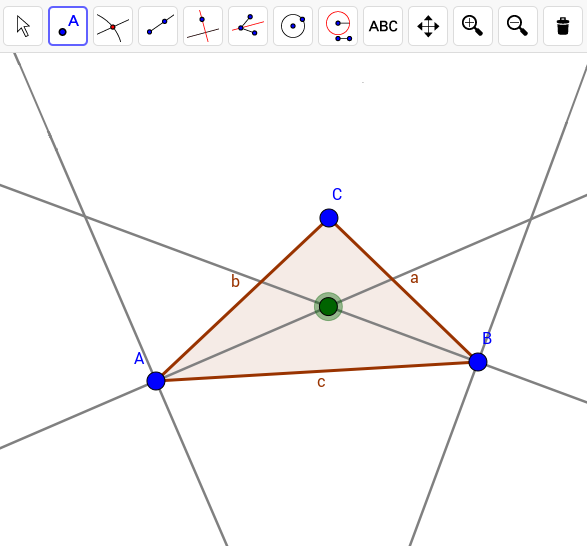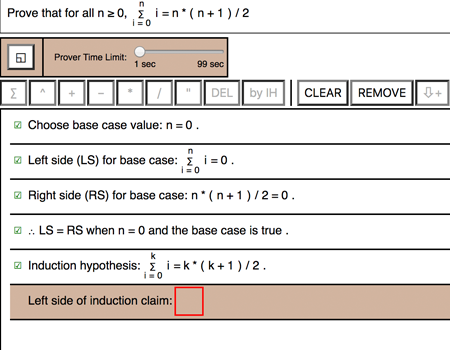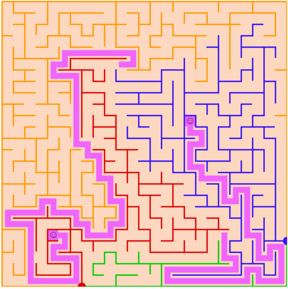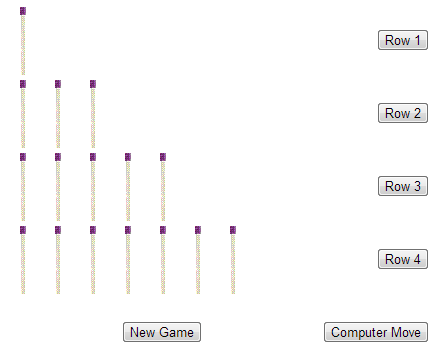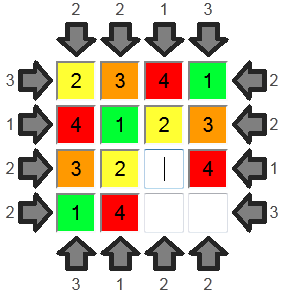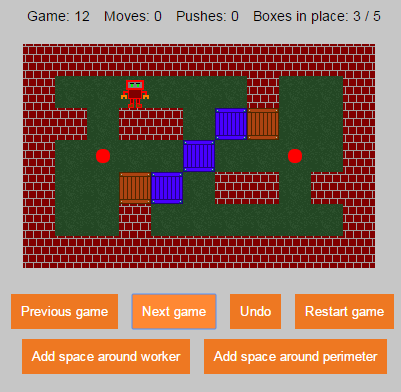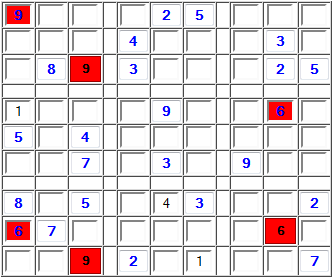## Today's Calcrostic Puzzle

```abc +   b = abd
× -   + +   -
b + abe = abc
= =   = =   =
bef / abc =   b
```
What is This? | How to Solve It?

## Yesterday's Solution

``` 2 + 21 = 23
× +  - +  -
21 - 19 =  2
= =  = =  =
42 /  2 = 21
```
Total number of plays of all games: 10,653,446